# RD Sharma Solutions for Class 11 Chapter 10 - Sine and Cosine Formulae and their Applications Exercise 10.2

This Exercise mainly focuses on problems based on the law of cosines, projection formulae, the law of tangents and area of a triangle. For students who face difficulty in solving problems, our faculty team works with the aim of making Mathematics easier for students, based on their grasping power. Students can have a strong command over the concepts covered in Maths Class 11 only by regular practice, and while solving problems can make use of the pdf available as reference material. Students can easily download the RD Sharma Class 11 Solutions for Maths Exercise 10.2 of Chapter 10 from the below-provided links.

## Download the Pdf of RD Sharma Solutions for Class 11 Maths Exercise 10.2 Chapter 10 – Sine and Cosine Formulae and their Applications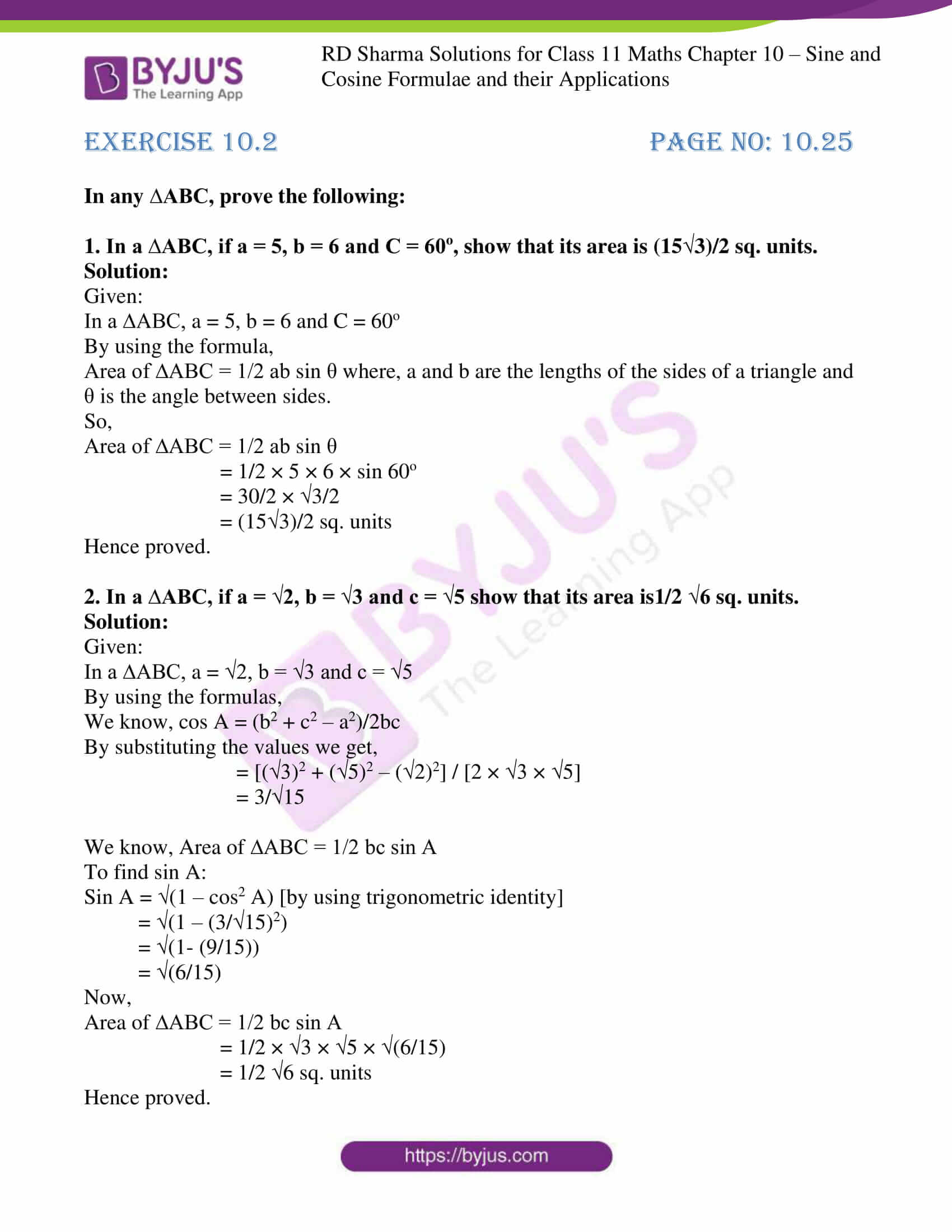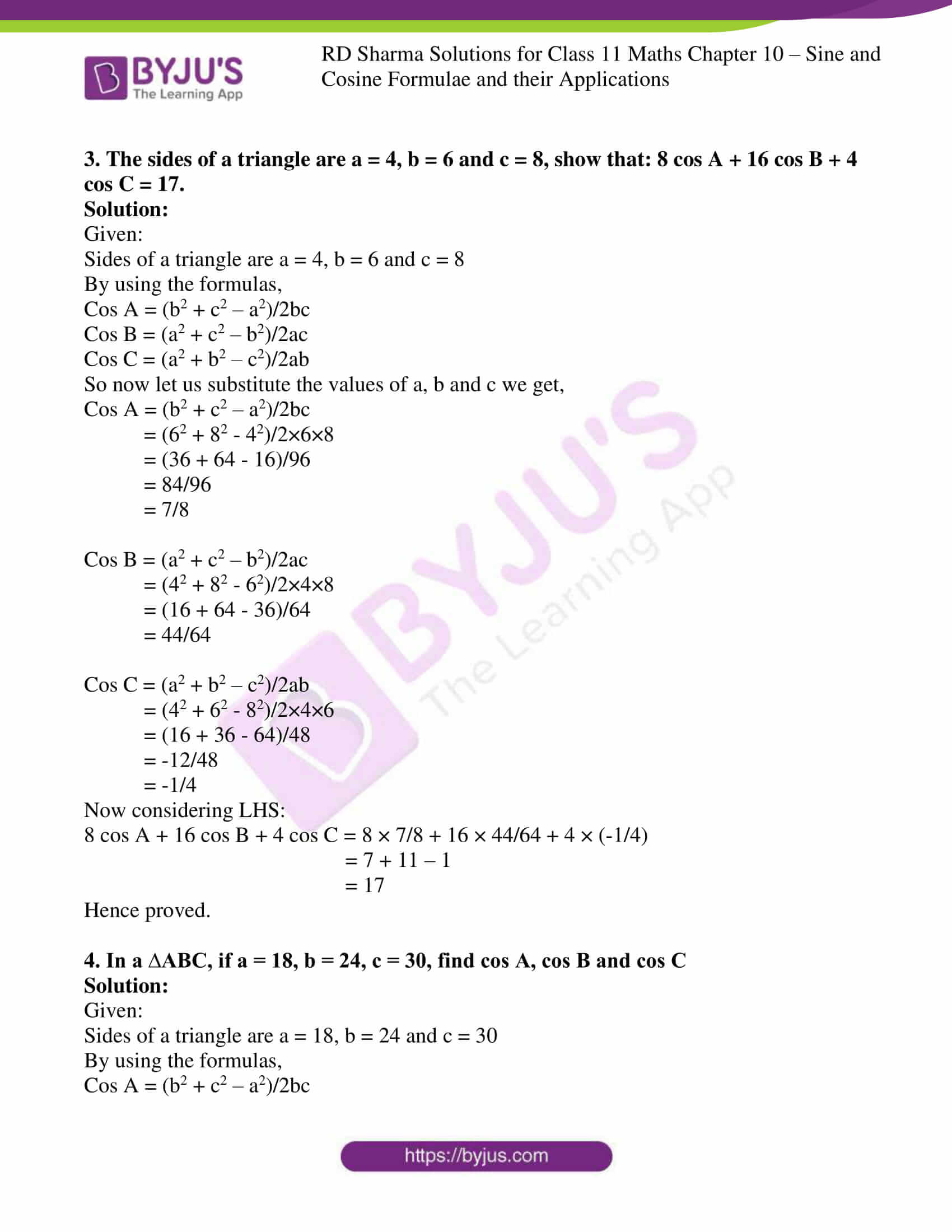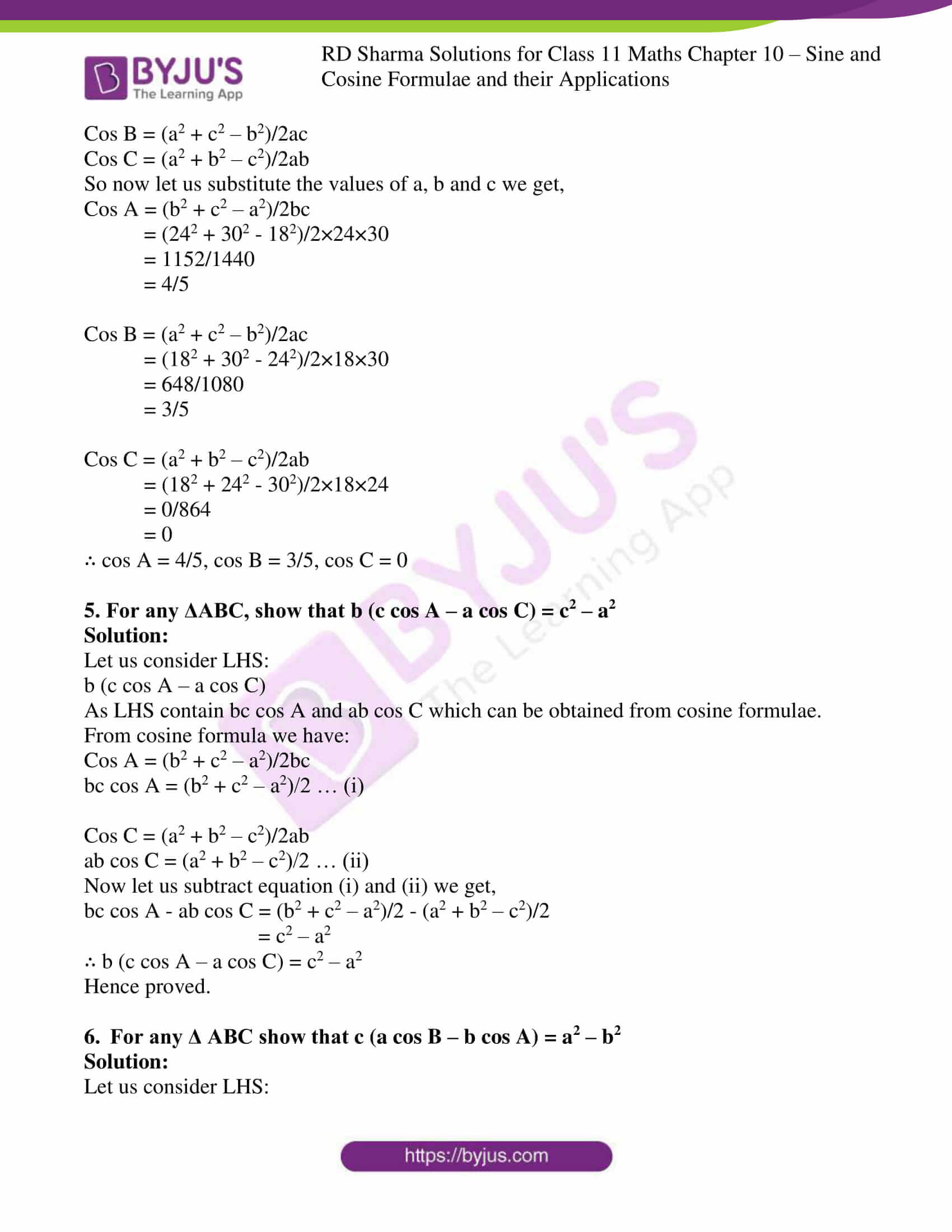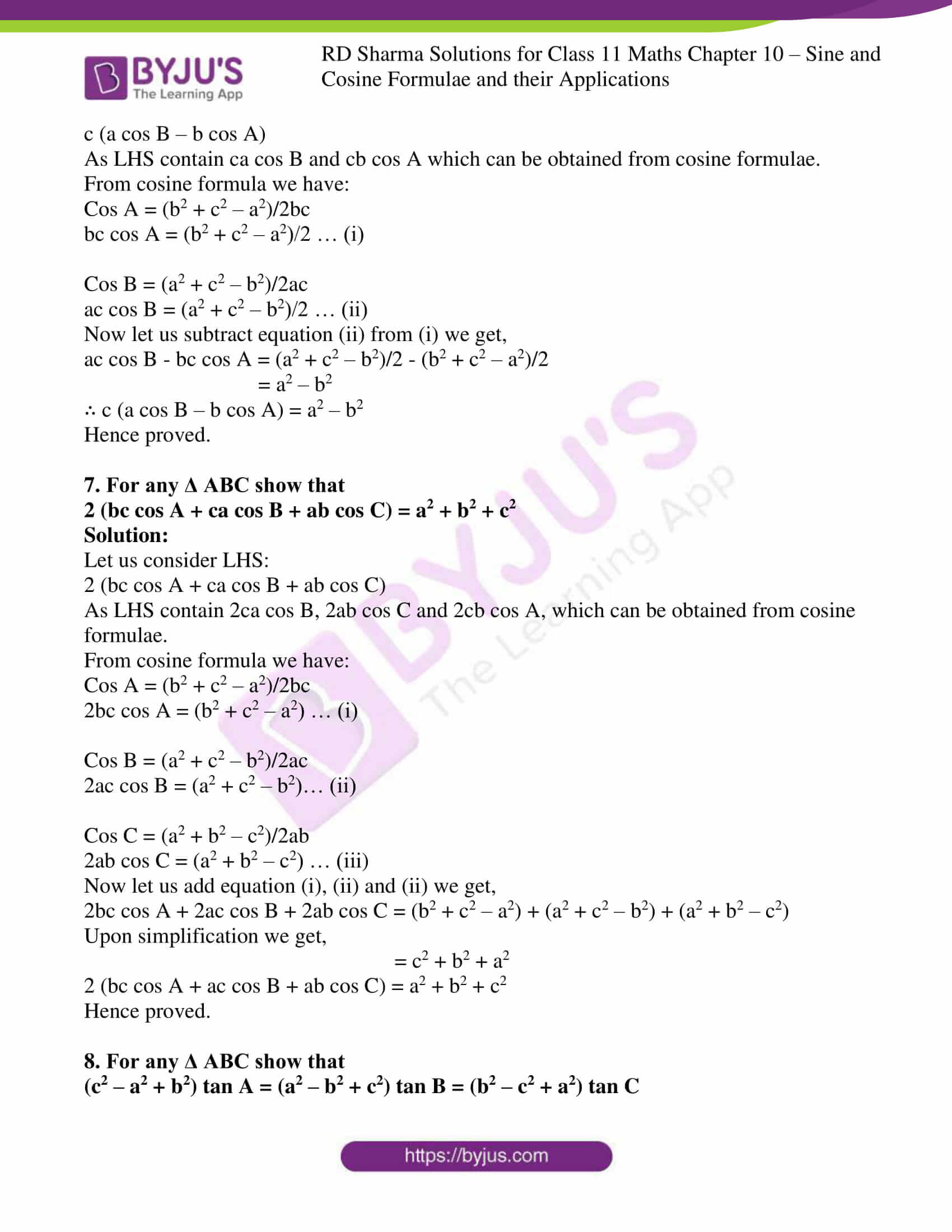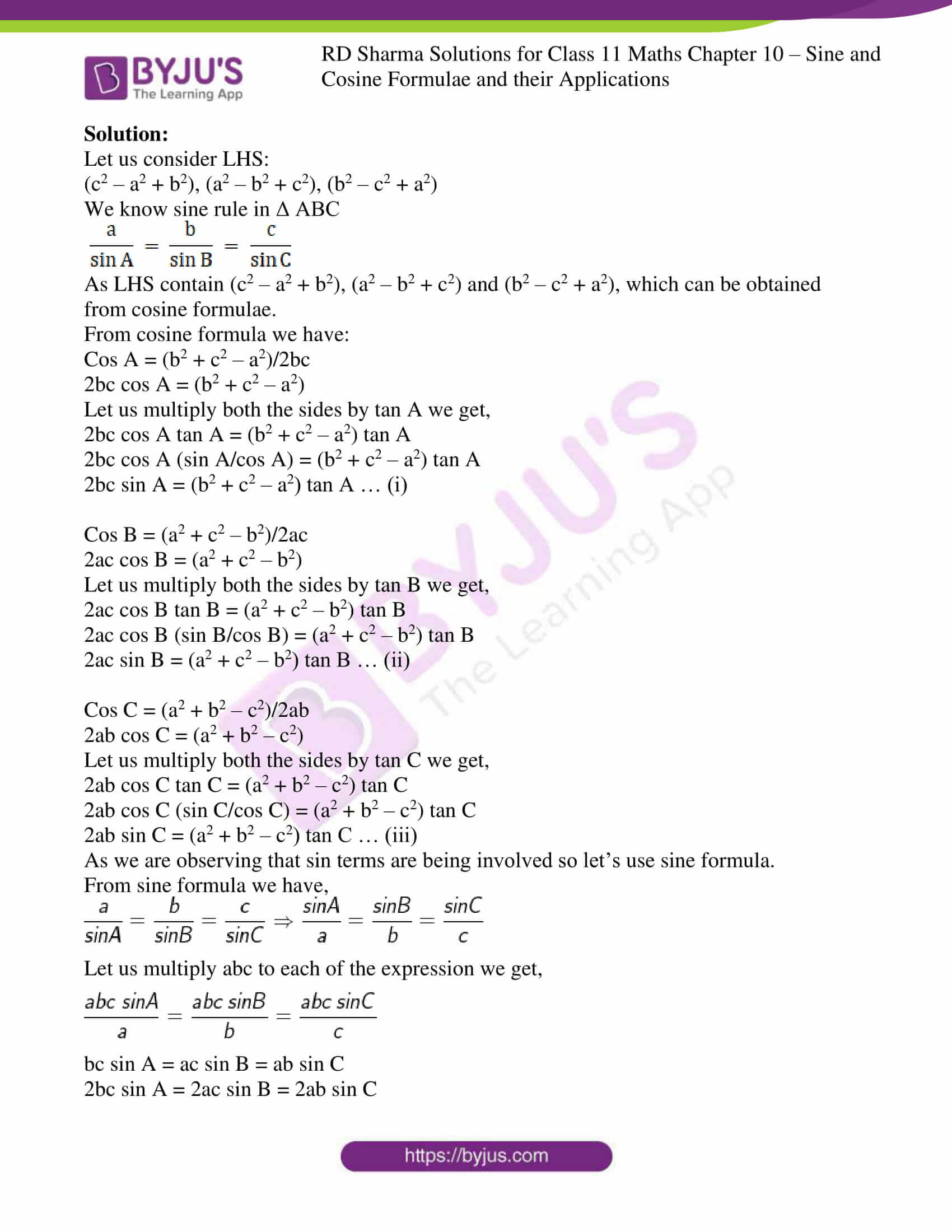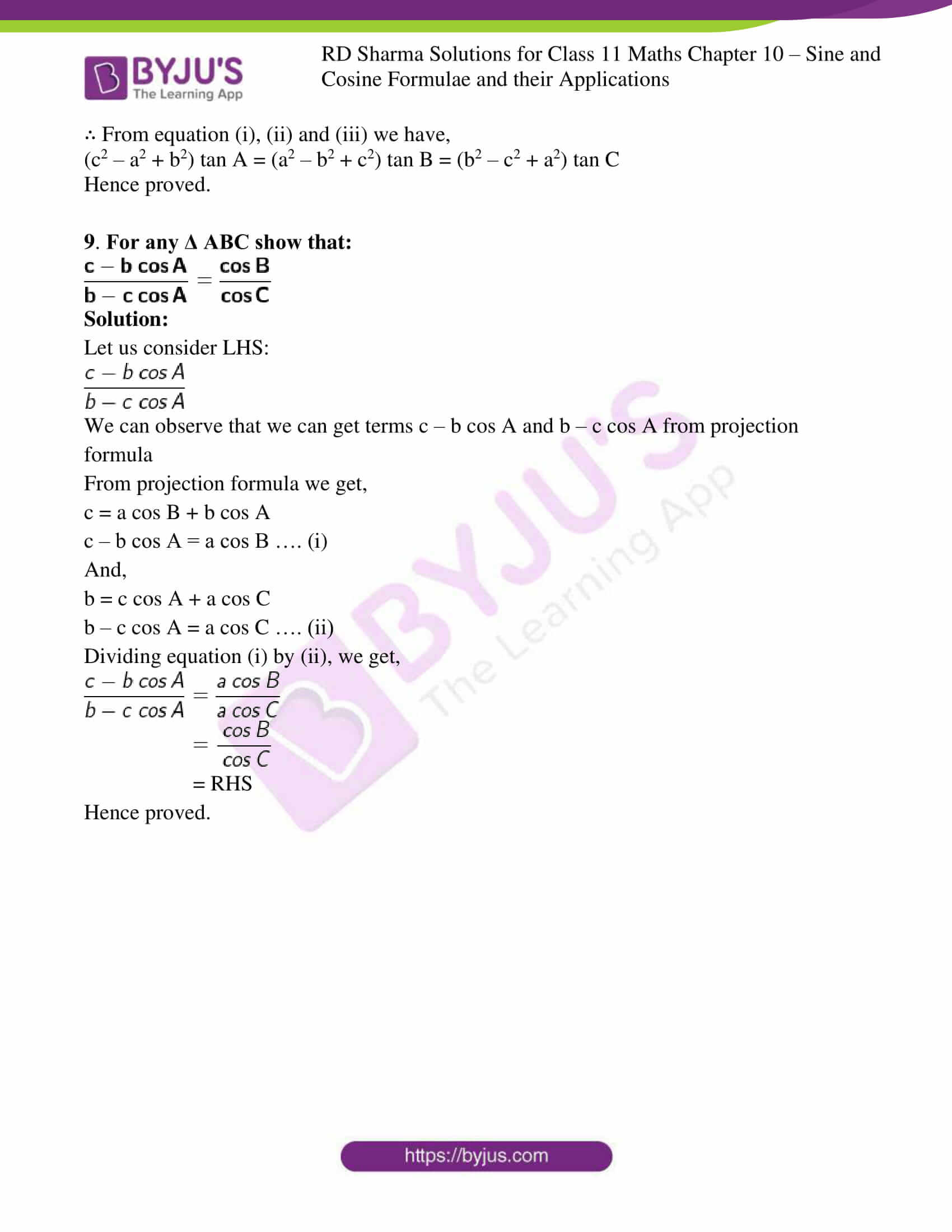### Access answers to RD Sharma Solutions for Class 11 Maths Exercise 10.2 Chapter 10 – Sine and Cosine Formulae and their Applications

In any ∆ABC, prove the following:

1. In a ∆ABC, if a = 5, b = 6 and C = 60o, show that its area is (15√3)/2 sq. units.

Solution:

Given:

In a ∆ABC, a = 5, b = 6 and C = 60o

By using the formula,

Area of ∆ABC = 1/2 ab sin θ where, a and b are the lengths of the sides of a triangle and θ is the angle between sides.

So,

Area of ∆ABC = 1/2 ab sin θ

= 1/2 × 5 × 6 × sin 60o

= 30/2 × 3/2

= (153)/2 sq. units

Hence proved.

2. In a ∆ABC, if a = √2, b = √3 and c = √5 show that its area is1/2 √6 sq. units.

Solution:

Given:

In a ∆ABC, a = √2, b = √3 and c = √5

By using the formulas,

We know, cos A = (b2 + c2 – a2)/2bc

By substituting the values we get,

= [(√3)2 + (√5)2 – (√2)2] / [2 × √3 × √5]

= 3/√15

We know, Area of ∆ABC = 1/2 bc sin A

To find sin A:

Sin A = √(1 – cos2 A) [by using trigonometric identity]

= √(1 – (3/√15)2)

= √(1- (9/15))

= √(6/15)

Now,

Area of ∆ABC = 1/2 bc sin A

= 1/2 × √3 × √5 × √(6/15)

= 1/2 √6 sq. units

Hence proved.

3. The sides of a triangle are a = 4, b = 6 and c = 8, show that: 8 cos A + 16 cos B + 4 cos C = 17.

Solution:

Given:

Sides of a triangle are a = 4, b = 6 and c = 8

By using the formulas,

Cos A = (b2 + c2 – a2)/2bc

Cos B = (a2 + c2 – b2)/2ac

Cos C = (a2 + b2 – c2)/2ab

So now let us substitute the values of a, b and c we get,

Cos A = (b2 + c2 – a2)/2bc

= (62 + 82 – 42)/2×6×8

= (36 + 64 – 16)/96

= 84/96

= 7/8

Cos B = (a2 + c2 – b2)/2ac

= (42 + 82 – 62)/2×4×8

= (16 + 64 – 36)/64

= 44/64

Cos C = (a2 + b2 – c2)/2ab

= (42 + 62 – 82)/2×4×6

= (16 + 36 – 64)/48

= -12/48

= -1/4

Now considering LHS:

8 cos A + 16 cos B + 4 cos C = 8 × 7/8 + 16 × 44/64 + 4 × (-1/4)

= 7 + 11 – 1

= 17

Hence proved.

4. In a ∆ABC, if a = 18, b = 24, c = 30, find cos A, cos B and cos C

Solution:

Given:

Sides of a triangle are a = 18, b = 24 and c = 30

By using the formulas,

Cos A = (b2 + c2 – a2)/2bc

Cos B = (a2 + c2 – b2)/2ac

Cos C = (a2 + b2 – c2)/2ab

So now let us substitute the values of a, b and c we get,

Cos A = (b2 + c2 – a2)/2bc

= (242 + 302 – 182)/2×24×30

= 1152/1440

= 4/5

Cos B = (a2 + c2 – b2)/2ac

= (182 + 302 – 242)/2×18×30

= 648/1080

= 3/5

Cos C = (a2 + b2 – c2)/2ab

= (182 + 242 – 302)/2×18×24

= 0/864

= 0

∴ cos A = 4/5, cos B = 3/5, cos C = 0

5. For any ΔABC, show that b (c cos A – a cos C) = c2 – a2

Solution:

Let us consider LHS:

b (c cos A – a cos C)

As LHS contain bc cos A and ab cos C which can be obtained from cosine formulae.

From cosine formula we have:

Cos A = (b2 + c2 – a2)/2bc

bc cos A = (b2 + c2 – a2)/2 … (i)

Cos C = (a2 + b2 – c2)/2ab

ab cos C = (a2 + b2 – c2)/2 … (ii)

Now let us subtract equation (i) and (ii) we get,

bc cos A – ab cos C = (b2 + c2 – a2)/2 – (a2 + b2 – c2)/2

= c2 – a2

∴ b (c cos A – a cos C) = c2 – a2

Hence proved.

6. For any Δ ABC show that c (a cos B – b cos A) = a2 – b2

Solution:

Let us consider LHS:

c (a cos B – b cos A)

As LHS contain ca cos B and cb cos A which can be obtained from cosine formulae.

From cosine formula we have:

Cos A = (b2 + c2 – a2)/2bc

bc cos A = (b2 + c2 – a2)/2 … (i)

Cos B = (a2 + c2 – b2)/2ac

ac cos B = (a2 + c2 – b2)/2 … (ii)

Now let us subtract equation (ii) from (i) we get,

ac cos B – bc cos A = (a2 + c2 – b2)/2 – (b2 + c2 – a2)/2

= a2 – b2

∴ c (a cos B – b cos A) = a2 – b2

Hence proved.

7. For any Δ ABC show that
2 (bc cos A + ca cos B + ab cos C) = a2 + b2 + c2

Solution:

Let us consider LHS:

2 (bc cos A + ca cos B + ab cos C)

As LHS contain 2ca cos B, 2ab cos C and 2cb cos A, which can be obtained from cosine formulae.

From cosine formula we have:

Cos A = (b2 + c2 – a2)/2bc

2bc cos A = (b2 + c2 – a2) … (i)

Cos B = (a2 + c2 – b2)/2ac

2ac cos B = (a2 + c2 – b2)… (ii)

Cos C = (a2 + b2 – c2)/2ab

2ab cos C = (a2 + b2 – c2) … (iii)

Now let us add equation (i), (ii) and (ii) we get,

2bc cos A + 2ac cos B + 2ab cos C = (b2 + c2 – a2) + (a2 + c2 – b2) + (a2 + b2 – c2)

Upon simplification we get,

= c2 + b2 + a2

2 (bc cos A + ac cos B + ab cos C) = a2 + b2 + c2

Hence proved.

8. For any Δ ABC show that
(c2 – a2 + b2) tan A = (a2 – b2 + c2) tan B = (b2 – c2 + a2) tan C

Solution:

Let us consider LHS:

(c2 – a2 + b2), (a2 – b2 + c2), (b2 – c2 + a2)

We know sine rule in Δ ABC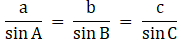As LHS contain (c2 – a2 + b2), (a2 – b2 + c2) and (b2 – c2 + a2), which can be obtained from cosine formulae.

From cosine formula we have:

Cos A = (b2 + c2 – a2)/2bc

2bc cos A = (b2 + c2 – a2)

Let us multiply both the sides by tan A we get,

2bc cos A tan A = (b2 + c2 – a2) tan A

2bc cos A (sin A/cos A) = (b2 + c2 – a2) tan A

2bc sin A = (b2 + c2 – a2) tan A … (i)

Cos B = (a2 + c2 – b2)/2ac

2ac cos B = (a2 + c2 – b2)

Let us multiply both the sides by tan B we get,

2ac cos B tan B = (a2 + c2 – b2) tan B

2ac cos B (sin B/cos B) = (a2 + c2 – b2) tan B

2ac sin B = (a2 + c2 – b2) tan B … (ii)

Cos C = (a2 + b2 – c2)/2ab

2ab cos C = (a2 + b2 – c2)

Let us multiply both the sides by tan C we get,

2ab cos C tan C = (a2 + b2 – c2) tan C

2ab cos C (sin C/cos C) = (a2 + b2 – c2) tan C

2ab sin C = (a2 + b2 – c2) tan C … (iii)

As we are observing that sin terms are being involved so let’s use sine formula.

From sine formula we have,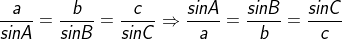Let us multiply abc to each of the expression we get,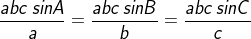bc sin A = ac sin B = ab sin C

2bc sin A = 2ac sin B = 2ab sin C

∴ From equation (i), (ii) and (iii) we have,

(c2 – a2 + b2) tan A = (a2 – b2 + c2) tan B = (b2 – c2 + a2) tan C

Hence proved.

9. For any Δ ABC show that: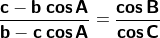Solution:

Let us consider LHS: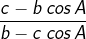We can observe that we can get terms c – b cos A and b – c cos A from projection formula

From projection formula we get,

c = a cos B + b cos A

c – b cos A = a cos B …. (i)

And,

b = c cos A + a cos C

b – c cos A = a cos C …. (ii)

Dividing equation (i) by (ii), we get,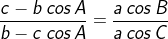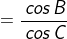= RHS

Hence proved.